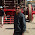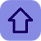# C Programming Data Type

Posted by Tushar BedekarIn C, variable(data) should be declared before it can be used in program. Data types are the keywords, which are used for assigning a type to a variable.In other words we can define the data type as a keyword which specifies the type of the data to be processed during the execution of the program me.

### Data types determine the following:• Range of the data
• Type of the data stored
• Number of bytes it occupies in memory

### C supports following data types:

• int – occupies 2 (16-bit compiler) or 4 bytes (32-bit compiler) of memory
• float – occupies 4 byes of memory
• double – occupies 8 bytes of memory
• char – occupies 1 byte of memory

### Integer Data Type:-

int is used to define integer numbers. It occupies 2 bytes of memory (16-bit compiler).Keyword int is used for declaring the variable with integer type. For example:
The size of int is either 2 bytes(In older PC's) or 4 bytes. If you consider an integer having size of 4 byte( equal to 32 bits), it can take 232 distinct states as: -231,-231+1, ...,-2, -1, 0, 1, 2, ..., 231-2, 231-1
Similarly, int of 2 bytes, it can take 216 distinct states from -215 to 215-1. If you try to store larger number than 231-1, i.e,+2147483647 and smaller number than -231, i.e, -2147483648,  program will not run correctly.

### Float Data Type:-

float is used to define floating type numbers. It occupies 4 bytes of memory
(16-bit compiler).Variables of floating types can hold real values(numbers) such as: 2.34, -9.382 etc. Keywords either float or double is used for declaring floating type variable. For example:

### Double Data Type:-

double is used to store big floating point numbers. It reserves 8 bytes of memory (16-bit compiler)

### Difference between float and double:-

Generally the size of float(Single precision float data type) is 4 bytes and that of double(Double precision float data type) is 8 bytes. Floating point variables has a precision of 6 digits whereas the the precision of double is 14 digits.
Note: Precision describes the number of significant decimal places that a floating values carries.

### Char Data Type:-

char is used to store characters. It occupies 1 byte of memory (16-bit compiler).The size of char is 1 byte. The character data type consists of ASCII characters. Each character is given a specific value. For example:

### Summary:

Following table is applicable for 16-bit compiler.

#### 1 comment:

1.Thanks for sharing ......